# Java Swing | JComboBox with examples

JComboBox is a part of Java Swing package. JComboBox inherits JComponent class . JComboBox shows a popup menu that shows a list and the user can select a option from that specified list . JComboBox can be editable or read- only depending on the choice of the programmer .

Constructor of the JComboBox are:

1. JComboBox() : creates a new empty JComboBox .
2. JComboBox(ComboBoxModel M) : creates a new JComboBox with items from specified ComboBoxModel
3. JComboBox(E [ ] i) : creates a new JComboBox with items from specified array.
4. JComboBox(Vector items) : creates a new JComboBox with items from the specified vector

Commonly used Methods are :

3. getItemAt(int i) : returns the item at index i
4. getItemCount(): returns the number of items from the list
5. getSelectedItem() : returns the item which is selected
6. removeItemAt(int i) : removes the element at index i
7. setEditable(boolean b) : the boolean b determines whether the combo box is editable or not .If true is passed then the combo box is editable or vice versa.
8. setSelectedIndex(int i): selects the element of JComboBox at index i.
9. showPopup() :causes the combo box to display its popup window.
10. setUI(ComboBoxUI ui): sets the L&F object that renders this component.
11. setSelectedItem(Object a): sets the selected item in the combo box display area to the object in the argument.
12. setSelectedIndex(int a): selects the item at index anIndex.
13. setPopupVisible(boolean v): sets the visibility of the popup.
14. setModel(ComboBoxModel a) : sets the data model that the JComboBox uses to obtain the list of items.
15. setMaximumRowCount(int count): sets the maximum number of rows the JComboBox displays.
16. setEnabled(boolean b): enables the combo box so that items can be selected.
17. removeItem(Object anObject) : removes an item from the item list.
18. removeAllItems(): removes all items from the item list.
19. removeActionListener(ActionListener l): removes an ActionListener.
20. isPopupVisible() : determines the visibility of the popup.
22. getActionCommand() : returns the action command that is included in the event sent to action listeners.
23. getEditor(): returns the editor used to paint and edit the selected item in the JComboBox field.
24. getItemCount() : returns the number of items in the list.
25. getItemListeners(): returns an array of all the ItemListeners added to this JComboBox with addItemListener().
26. createDefaultKeySelectionManager() : returns an instance of the default key-selection manager.
27. fireItemStateChanged(ItemEvent e) : notifies all listeners that have registered interest for notification on this event type.
28. firePopupMenuCanceled() : notifies PopupMenuListeners that the popup portion of the combo box has been canceled.
29. firePopupMenuWillBecomeInvisible() : notifies PopupMenuListeners that the popup portion of the combo box has become invisible.
30. firePopupMenuWillBecomeVisible() : notifies PopupMenuListeners that the popup portion of the combo box will become visible.
31. setEditor(ComboBoxEditor a): sets the editor used to paint and edit the selected item in the JComboBox field.
32. setActionCommand(String a) : sets the action command that should be included in the event sent to actionListeners.
33. getUI() : returns the look and feel object that renders this component.
34. paramString() : returns a string representation of this JComboBox.
35. getUIClassID() : returns the name of the Look and feel class that renders this component.
36. getAccessibleContext() : gets the AccessibleContext associated with this JComboBox

The following programs will illustrate the use of JComboBox

1. Program to create a simple JComboBox and add elements to it .

 `// Java Program to create a simple JComboBox  ` `// and add elements to it ` `import` `java.awt.event.*; ` `import` `java.awt.*; ` `import` `javax.swing.*; ` `class` `solve ``extends` `JFrame ``implements` `ItemListener { ` ` `  `    ``// frame ` `    ``static` `JFrame f; ` ` `  `    ``// label ` `    ``static` `JLabel l, l1; ` ` `  `    ``// combobox ` `    ``static` `JComboBox c1; ` ` `  `    ``// main class ` `    ``public` `static` `void` `main(String[] args) ` `    ``{ ` `        ``// create a new frame ` `        ``f = ``new` `JFrame(``"frame"``); ` ` `  `        ``// create a object ` `        ``solve s = ``new` `solve(); ` ` `  `        ``// set layout of frame ` `        ``f.setLayout(``new` `FlowLayout()); ` ` `  `        ``// array of string contating cities ` `        ``String s1[] = { ``"Jalpaiguri"``, ``"Mumbai"``, ``"Noida"``, ``"Kolkata"``, ``"New Delhi"` `}; ` ` `  `        ``// create checkbox ` `        ``c1 = ``new` `JComboBox(s1); ` ` `  `        ``// add ItemListener ` `        ``c1.addItemListener(s); ` ` `  `        ``// create labels ` `        ``l = ``new` `JLabel(``"select your city "``); ` `        ``l1 = ``new` `JLabel(``"Jalpaiguri selected"``); ` ` `  `        ``// set color of text ` `        ``l.setForeground(Color.red); ` `        ``l1.setForeground(Color.blue); ` ` `  `        ``// create a new panel ` `        ``JPanel p = ``new` `JPanel(); ` ` `  `        ``p.add(l); ` ` `  `        ``// add combobox to panel ` `        ``p.add(c1); ` ` `  `        ``p.add(l1); ` ` `  `        ``// add panel to frame ` `        ``f.add(p); ` ` `  `        ``// set the size of frame ` `        ``f.setSize(``400``, ``300``); ` ` `  `        ``f.show(); ` `    ``} ` `    ``public` `void` `itemStateChanged(ItemEvent e) ` `    ``{ ` `        ``// if the state combobox is changed ` `        ``if` `(e.getSource() == c1) { ` ` `  `            ``l1.setText(c1.getSelectedItem() + ``" selected"``); ` `        ``} ` `    ``} ` `} `

Output :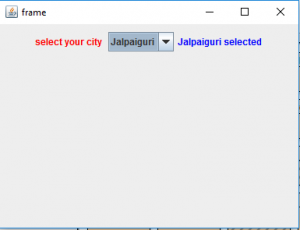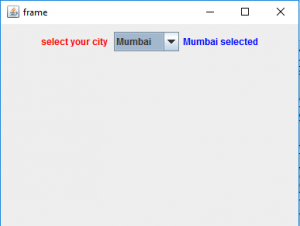2. Program to create two checkbox one editable and other read only

 `// Java Program to create two  checkbox  ` `// one editable and other read only ` `import` `java.awt.event.*; ` `import` `java.awt.*; ` `import` `javax.swing.*; ` `class` `solve ``extends` `JFrame ``implements` `ItemListener { ` ` `  `    ``// frame ` `    ``static` `JFrame f; ` ` `  `    ``// label ` `    ``static` `JLabel l, l1, l3, l4; ` ` `  `    ``// combobox ` `    ``static` `JComboBox c1, c2; ` ` `  `    ``// main class ` `    ``public` `static` `void` `main(String[] args) ` `    ``{ ` `        ``// create a new frame ` `        ``f = ``new` `JFrame(``"frame"``); ` ` `  `        ``// create a object ` `        ``solve s = ``new` `solve(); ` ` `  `        ``// array of string contating cities ` `        ``String s1[] = { ``"Jalpaiguri"``, ``"Mumbai"``, ``"Noida"``, ``"Kolkata"``, ``"New Delhi"` `}; ` `        ``String s2[] = { ``"male"``, ``"female"``, ``"others"` `}; ` ` `  `        ``// create checkbox ` `        ``c1 = ``new` `JComboBox(s1); ` `        ``c2 = ``new` `JComboBox(s2); ` ` `  `        ``// set Kolakata and male as selected items ` `        ``// using setSelectedIndex ` `        ``c1.setSelectedIndex(``3``); ` `        ``c2.setSelectedIndex(``0``); ` ` `  `        ``// add ItemListener ` `        ``c1.addItemListener(s); ` `        ``c2.addItemListener(s); ` ` `  `        ``// set the checkbox as editable ` `        ``c1.setEditable(``true``); ` ` `  `        ``// create labels ` `        ``l = ``new` `JLabel(``"select your city "``); ` `        ``l1 = ``new` `JLabel(``"Jalpaiguri selected"``); ` `        ``l3 = ``new` `JLabel(``"select your gender "``); ` `        ``l4 = ``new` `JLabel(``"Male selected"``); ` ` `  `        ``// set color of text ` `        ``l.setForeground(Color.red); ` `        ``l1.setForeground(Color.blue); ` `        ``l3.setForeground(Color.red); ` `        ``l4.setForeground(Color.blue); ` ` `  `        ``// create a new panel ` `        ``JPanel p = ``new` `JPanel(); ` ` `  `        ``p.add(l); ` ` `  `        ``// add combobox to panel ` `        ``p.add(c1); ` ` `  `        ``p.add(l1); ` ` `  `        ``p.add(l3); ` ` `  `        ``// add combobox to panel ` `        ``p.add(c2); ` ` `  `        ``p.add(l4); ` ` `  `        ``// set a layout for panel ` `        ``p.setLayout(``new` `FlowLayout()); ` ` `  `        ``// add panel to frame ` `        ``f.add(p); ` ` `  `        ``// set the size of frame ` `        ``f.setSize(``400``, ``400``); ` ` `  `        ``f.show(); ` `    ``} ` `    ``public` `void` `itemStateChanged(ItemEvent e) ` `    ``{ ` `        ``// if the state combobox 1is changed ` `        ``if` `(e.getSource() == c1) { ` ` `  `            ``l1.setText(c1.getSelectedItem() + ``" selected"``); ` `        ``} ` ` `  `        ``// if state of combobox 2 is changed ` `        ``else` `            ``l4.setText(c2.getSelectedItem() + ``" selected"``); ` `    ``} ` `} `

Output :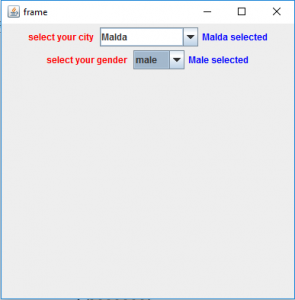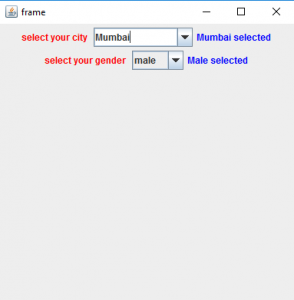3. Program to create a checkbox and add or remove items from it .

 `// Java  Program to create a checkbox  ` `// and add or remove items from it ` `import` `java.awt.event.*; ` `import` `java.awt.*; ` `import` `javax.swing.*; ` `class` `solve11 ``extends` `JFrame ``implements` `ItemListener, ActionListener { ` ` `  `    ``// frame ` `    ``static` `JFrame f; ` ` `  `    ``// label ` `    ``static` `JLabel l, l1; ` ` `  `    ``// combobox ` `    ``static` `JComboBox c1; ` ` `  `    ``// textfield to add and delete items ` `    ``static` `JTextField tf; ` ` `  `    ``// main class ` `    ``public` `static` `void` `main(String[] args) ` `    ``{ ` `        ``// create a new frame ` `        ``f = ``new` `JFrame(``"frame"``); ` ` `  `        ``// create a object ` `        ``solve11 s = ``new` `solve11(); ` ` `  `        ``// set layout of frame ` `        ``f.setLayout(``new` `FlowLayout()); ` ` `  `        ``// array of string contating cities ` `        ``String s1[] = { ``"Jalpaiguri"``, ``"Mumbai"``, ``"Noida"``, ``"Kolkata"``, ``"New Delhi"` `}; ` ` `  `        ``// create checkbox ` `        ``c1 = ``new` `JComboBox(s1); ` ` `  `        ``// create textfield ` `        ``tf = ``new` `JTextField(``16``); ` ` `  `        ``// create add and remove buttons ` `        ``JButton b = ``new` `JButton(``"ADD"``); ` `        ``JButton b1 = ``new` `JButton(``"REMOVE"``); ` ` `  `        ``// add action listener ` `        ``b.addActionListener(s); ` `        ``b1.addActionListener(s); ` ` `  `        ``// add ItemListener ` `        ``c1.addItemListener(s); ` ` `  `        ``// create labels ` `        ``l = ``new` `JLabel(``"select your city "``); ` `        ``l1 = ``new` `JLabel(``"Jalpaiguri selected"``); ` ` `  `        ``// set color of text ` `        ``l.setForeground(Color.red); ` `        ``l1.setForeground(Color.blue); ` ` `  `        ``// create a new panel ` `        ``JPanel p = ``new` `JPanel(); ` ` `  `        ``p.add(l); ` ` `  `        ``// add combobox to panel ` `        ``p.add(c1); ` ` `  `        ``p.add(l1); ` `        ``p.add(tf); ` `        ``p.add(b); ` `        ``p.add(b1); ` ` `  `        ``f.setLayout(``new` `FlowLayout()); ` ` `  `        ``// add panel to frame ` `        ``f.add(p); ` ` `  `        ``// set the size of frame ` `        ``f.setSize(``700``, ``200``); ` ` `  `        ``f.show(); ` `    ``} ` `    ``// if button is pressed ` `    ``public` `void` `actionPerformed(ActionEvent e) ` `    ``{ ` `        ``String s = e.getActionCommand(); ` `        ``if` `(s.equals(``"ADD"``)) { ` `            ``c1.addItem(tf.getText()); ` `        ``} ` `        ``else` `{ ` `            ``c1.removeItem(tf.getText()); ` `        ``} ` `    ``} ` ` `  `    ``public` `void` `itemStateChanged(ItemEvent e) ` `    ``{ ` `        ``// if the state combobox is changed ` `        ``if` `(e.getSource() == c1) { ` ` `  `            ``l1.setText(c1.getSelectedItem() + ``" selected"``); ` `        ``} ` `    ``} ` `} `

Output :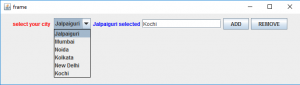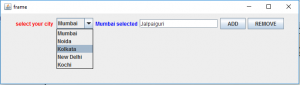Note : the above programs might not run in an online compiler please use an offline IDE

Attention reader! Don’t stop learning now. Get hold of all the important Java and Collections concepts with the Fundamentals of Java and Java Collections Course at a student-friendly price and become industry ready.

My Personal Notes arrow_drop_upThird year Department of Information Technology Jadavpur University

If you like GeeksforGeeks and would like to contribute, you can also write an article using contribute.geeksforgeeks.org or mail your article to contribute@geeksforgeeks.org. See your article appearing on the GeeksforGeeks main page and help other Geeks.

Please Improve this article if you find anything incorrect by clicking on the "Improve Article" button below.

Article Tags :
Practice Tags :

1

Please write to us at contribute@geeksforgeeks.org to report any issue with the above content.